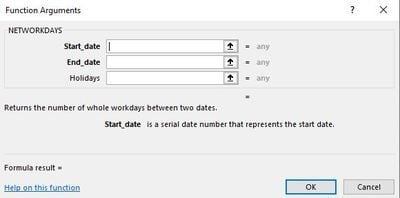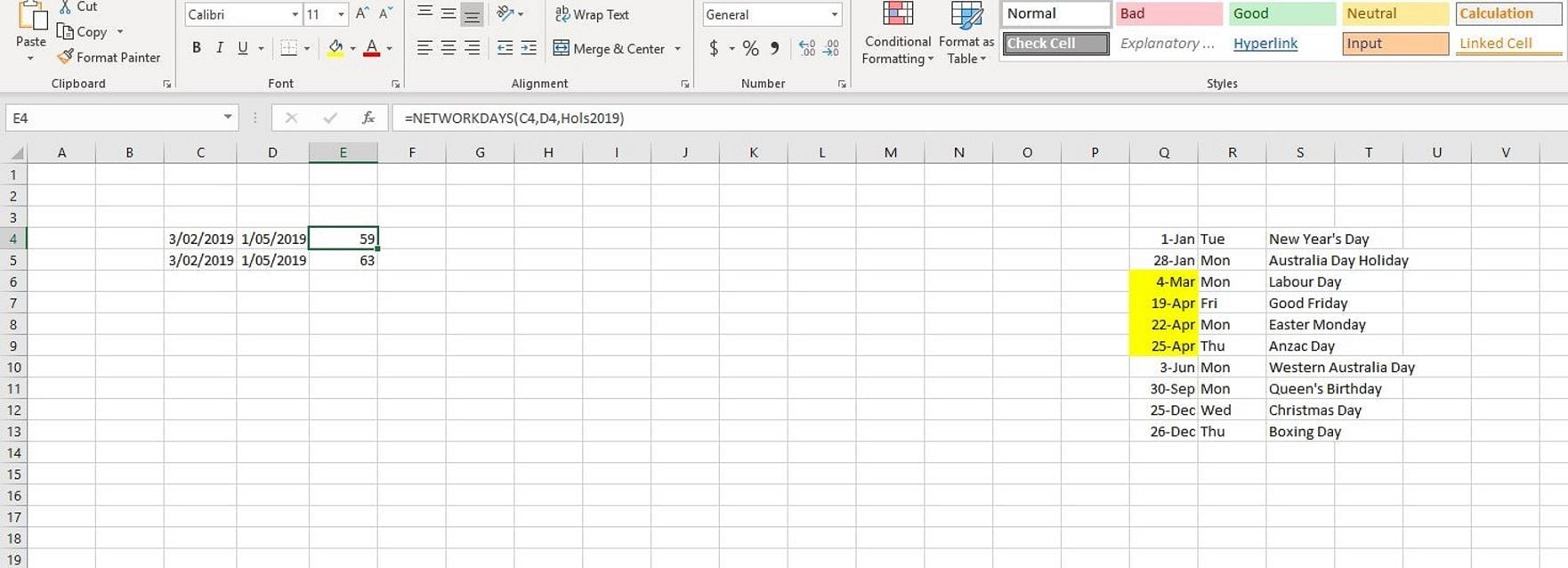Home >  Blog >  Calculate working days in Excel

# Calculate working days in Excel

Posted by Datasift on 9 March 2019

Often in Excel models we need to calculate the number of working days between two dates.

For example we have a sales figure generated between two dates and we want to calculate the sales per day so

that we can test our forecast for reasonableness.

For this we can use the function NETWORKDAYS.The function has three components - Start_date and End_Date are fairly obvious, but what is the Holidays component?

It is simply a list of days that you need excluded from the calculation, for example public holidays.

In the example below, we have used a list of public holidays and created a named range called Hols2019 across cells Q4:Q13

By including the range in the formula, those dates that fall between our start and end dates are excluded to give an answer of 59 days,

Whereas in the second line we have just used start and end dates to get an answer of  63 days.Author: Datasift
 Tags: Top Tip